# 离心力对轴对称弯道河槽张力作用的研究Study on the Effect of Centrifugal Force on River Channel Tension in Axisymmetric Bend

DOI: 10.12677/APP.2019.911056, PDF, HTML, XML, 下载: 1,132  浏览: 3,943

Abstract: A uniform circular motion system model with symmetrical mirror image is adopted according to the action and reaction properties of centripetal force and centrifugal force. Under the action of constrained centrifugal force, the whole system generates reciprocating vibration. It is applied to the calculation of the effect of the centrifugal force on the tension of the river bed. It can be applied to the design of circular arc water conservancy projects and flood control safety.

1. 引言

2. 圆周运动物体系统的向心力与离心力是作用力与反作用力的依据

3. 匀速圆周运动物体系统在受约束离心力作用下产生周期性运动

3.1. 构建物理实验模型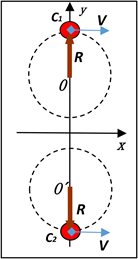Figure 1. Mirror symmetrical circular motion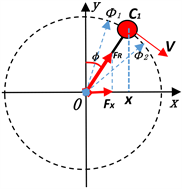Figure 2. Centrifugal force analysis

3.2. 匀速圆周运动系统离心力作用的解析

3.2.1. 匀速圆周运动系统离心力及在x轴方向上的冲量

${F}_{R}=-{{F}^{\prime }}_{R},$

${F}_{x}={F}_{R}\mathrm{sin}\varphi =-{{F}^{\prime }}_{R}\mathrm{sin}\varphi =-m\frac{{v}^{2}}{R}\mathrm{sin}\varphi$.

$\because {v}_{x}=v\mathrm{cos}\varphi$${v}_{{x}_{1}}=v\mathrm{cos}{\varphi }_{1}$${v}_{{x}_{2}}=v\mathrm{cos}{\varphi }_{2}$$t=\frac{R\varphi }{v}$

$\begin{array}{l}\therefore {\int }_{{t}_{1}}^{{t}_{2}}{F}_{x}\text{d}t={\stackrel{¯}{F}}_{x}\left({t}_{2}-{t}_{1}\right)={\int }_{{t}_{1}}^{{t}_{2}}\left(-m\frac{{v}^{2}}{R}\right)\mathrm{sin}\varphi \text{d}t={\int }_{{\varphi }_{1}}^{{\varphi }_{2}}\left(-m\frac{{v}^{2}}{R}\right)\mathrm{sin}\varphi \text{d}\left(\frac{R\varphi }{v}\right)\\ \text{\hspace{0.17em}}\text{\hspace{0.17em}}\text{\hspace{0.17em}}\text{\hspace{0.17em}}\text{\hspace{0.17em}}\text{\hspace{0.17em}}\text{\hspace{0.17em}}\text{\hspace{0.17em}}\text{\hspace{0.17em}}\text{\hspace{0.17em}}\text{\hspace{0.17em}}\text{\hspace{0.17em}}\text{\hspace{0.17em}}=mv\mathrm{cos}{\varphi }_{2}-mv\mathrm{cos}{\varphi }_{1}=M{v}_{2\left(M\right)}-M{v}_{1\left(M\right)}\end{array}$

${\stackrel{¯}{F}}_{x}=\frac{mv\mathrm{cos}{\varphi }_{2}-mv\mathrm{cos}{\varphi }_{1}}{{t}_{2}-{t}_{1}}$.

${v}_{2\left(M\right)}=\frac{mv\mathrm{cos}{\varphi }_{2}-mv\mathrm{cos}{\varphi }_{1}+M{v}_{1\left(M\right)}}{M}$

${\int }_{0}^{\frac{T}{2}}{F}_{x}\text{d}t={\stackrel{¯}{F}}_{x}\frac{T}{2}={\stackrel{¯}{F}}_{x}\frac{\pi R}{v}={mv\mathrm{cos}{\varphi }_{2}-mv\mathrm{cos}{\varphi }_{1}|}_{0}^{\pi }=-2mv$

${\stackrel{¯}{F}}_{x}=\frac{mv\mathrm{cos}{\varphi }_{2}-mv\mathrm{cos}{\varphi }_{1}}{{t}_{2}-{t}_{1}}=\frac{-2m{v}^{2}}{\pi R}$.

${v}_{1\left(M\right)}=0$，且 $M=km$

${v}_{2\left(M\right)}=\frac{mv\mathrm{cos}{\varphi }_{2}-mv\mathrm{cos}{\varphi }_{1}}{km}=\frac{-2mv}{km}=-\frac{2}{k}v.$

$k=3$${v}_{2\left(M\right)}=-\frac{2}{3}v$$k=2$${v}_{2\left(M\right)}=-v$

${\int }_{\frac{T}{2}}^{T}{F}_{x}\text{d}t={\stackrel{¯}{F}}_{x}\frac{T}{2}={\stackrel{¯}{F}}_{x}\frac{\pi R}{v}={mv\mathrm{cos}{\varphi }_{2}-mv\mathrm{cos}{\varphi }_{1}|}_{\pi }^{2\pi }=2mv$

${\stackrel{¯}{F}}_{x}=\frac{mv\mathrm{cos}{\varphi }_{3}-mv\mathrm{cos}{\varphi }_{2}}{{t}_{3}-{t}_{2}}=\frac{2m{v}^{2}}{\pi R}$.

3.2.2. 离心力在x轴上的分量所做的功

$\begin{array}{c}{\int }_{{x}_{1}}^{{x}_{2}}{F}_{x}\text{d}x={\stackrel{¯}{F}}_{x}\left({x}_{2}-{x}_{1}\right)={\int }_{{x}_{1}}^{{x}_{2}}\left(-m\frac{{v}^{2}}{R}\right)\mathrm{sin}\varphi \text{d}x\\ ={\int }_{{x}_{1}}^{{x}_{2}}\left(-m\frac{{v}^{2}}{R}\right)\mathrm{sin}\varphi \text{d}\left(R\mathrm{sin}\varphi \right)\\ =\frac{1}{2}m{v}^{2}{\mathrm{cos}}^{2}{\varphi }_{2}-\frac{1}{2}m{v}^{2}{\mathrm{cos}}^{2}{\varphi }_{1}\\ =\frac{1}{2}M{v}_{2\left(M\right)}^{2}-\frac{1}{2}M{v}_{1\left(M\right)}^{2}\end{array}$

$W={\int }_{a}^{b}|f\left(x\right)\text{d}x|=±{\int }_{a}^{b}f\left(x\right)\text{d}x$

${C}_{1}$ 在0→π区间的转动，相对应的系统A速度为 ${v}_{0\left(M\right)}\to {v}_{M}$。根据参考文献    和分析研究，离心力在前半周(0→π/2)、(π/2→π)做正功，假设系统机械能守恒：

$\begin{array}{c}{W|}_{0}^{\pi }={\int }_{0}^{\frac{\pi }{2}}|{{F}^{\prime }}_{x}\text{d}x|+{\int }_{\frac{\pi }{2}}^{\pi }|{{F}^{\prime }}_{x}\text{d}x|\\ =\frac{1}{2}m{v}^{2}+\frac{1}{2}m{v}^{2}=\frac{1}{2}M{v}_{M}^{2}-\frac{1}{2}M{v}_{0\left(M\right)}^{2}\end{array}$

$M=km$，假设 ${v}_{0\left(M\right)}=0$

$\begin{array}{c}{\int }_{{x}_{1}}^{{x}_{2}}{F}_{x}\text{d}x={\stackrel{¯}{F}}_{x}\left[{x}_{2\left(M\right)}-{x}_{1\left(M\right)}\right]={\stackrel{¯}{F}}_{x}{X}_{M}=m{v}^{2}\\ =\frac{1}{2}M{v}_{2\left(M\right)}^{2}=\frac{1}{2}km{\left(-\frac{2v}{k}\right)}^{2}=\frac{2}{k}m{v}^{2}，\end{array}$

${X}_{M}=\frac{\frac{1}{2}M{v}_{2\left(M\right)}^{2}}{{\stackrel{¯}{F}}_{x}}=\frac{2}{k}m{v}^{2}\frac{\pi R}{2m{v}^{2}}=\frac{\pi R}{k}$

${X}_{M}=\frac{\pi R}{2}$.

3.2.3. 后半周(π→2π)区间离心力在x轴上的分量做的功和振幅

$\begin{array}{c}{|W||}_{\pi }^{2\pi }={\int }_{\pi }^{\frac{3\pi }{2}}|{{F}^{\prime }}_{x}\text{d}x|+{\int }_{\frac{3\pi }{2}}^{2\pi }|{{F}^{\prime }}_{x}\text{d}x|\\ =\frac{1}{2}m{v}^{2}+\frac{1}{2}m{v}^{2}=-\left[\frac{1}{2}M{v}_{{M}_{3}}^{2}-\frac{1}{2}M{v}_{M}^{2}\right].\end{array}$

${\stackrel{¯}{F}}_{x}=\frac{mv\mathrm{cos}{\varphi }_{3}-mv\mathrm{cos}{\varphi }_{2}}{{t}_{3}-{t}_{2}}=\frac{-2m{v}^{2}}{\pi R}$.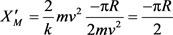。(同上半周)

3.2.4. 前后半周(0→2π)做功之和

$W={W|}_{0}^{\pi }-{|W||}_{\pi }^{2\pi }=0$.

4. 轴对称  圆弧弯道水流离心力对河道张力作用的解析算法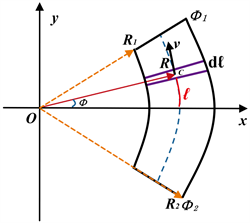Figure 3. Schematic diagram of arc curve river channel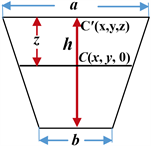Figure 4. River cross section

$M=Nm=n\mathcal{l}m$

$\begin{array}{c}{\int }_{-\mathcal{l}}^{\mathcal{l}}{F}_{x}n\text{d}\mathcal{l}=n{\int }_{{\varphi }_{1}}^{{\varphi }_{2}}{{F}^{\prime }}_{R}\mathrm{cos}\varphi \text{d}\left(R\varphi \right)=n{\int }_{{\varphi }_{1}}^{{\varphi }_{2}}m\frac{{v}^{2}}{R}\mathrm{cos}\varphi \text{d}\left(R\varphi \right)\\ =\frac{M}{\mathcal{l}}{v}^{2}\left(\mathrm{sin}{\varphi }_{2}-\mathrm{sin}{\varphi }_{1}\right)=2\frac{M}{\mathcal{l}}{v}^{2}\mathrm{sin}{\varphi }_{2}.\end{array}$

2012年7月24日长江三峡发生7万m3洪峰流量的罕见洪水，夹杂着泥沙直扑三峡大坝。如流经圆弧河槽段，平均河宽1000 m，平均河深5 m，圆弧河段有效长1000 m，有效半径5000 m， $\text{2}\varphi ={\text{17}}^{\circ }\text{27'}$，平均流速14 m/s，该段圆弧弯道水流沿x轴方向对河槽的张力：

${\int }_{-\mathcal{l}}^{\mathcal{l}}{F}_{x}n\text{d}\mathcal{l}=n{\int }_{{\varphi }_{1}}^{{\varphi }_{2}}{{F}^{\prime }}_{R}\mathrm{cos}\varphi \text{d}\left(R\varphi \right)=2\frac{M}{\mathcal{l}}{v}^{2}\mathrm{sin}{\varphi }_{2}=195673.5\text{\hspace{0.17em}}\text{N}=\text{19}\text{.57}\left(吨\right)$

5. 结论

  李泽华. 人造卫星运行中的向心力和离心力问题[J]. 物理通报, 1962(3): 132-136.  谢仁荣. 解读人造卫星运行中的向心力和离心力问题[J]. 数理化解题研究, 2012(I): 32-34.  孙璐. 大角度受控离心力对系统的无工质推进方法研究[J]. 科技创新导报, 2019(21): 49-52.  孙璐. 电磁驱动式无工质牵引器[P]. 中国201721222213.4, 2017-09-22.  孙璐, 等. 内力用于系统的无工质自主推进方法研究[C]//中国电工技术学会电磁发射技术专业委员会. 全国第八届电磁发射技术研讨会论文集: 2017 年卷. 郑州, 2017: 198-204.  孙璐, 等. 轴对称线圈磁场的精确计算方法研究[J]. 军械工程学院学报, 2016, 28(3): 71-78.  王志鹏. 浅析河流弯道水流特点及冲刷深度[J]. 农业科技与信息, 2018(23): 123-124.  刘庆华, 李道霞. 弯道水流浅析[J]. 天中学刊, 1998(5): 120.  戴文鸿, 苗伟波, 高嵩, 等. 弯道水流运动及床面变形数值模拟研究进展[J]. 南水北调与水利科技, 2014(3): 121-124.  刘健, 郭维东. 弯道河段水流流态试验研究[J]. 人民长江, 2008(16): 81-84.